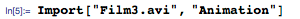Browse by Topic
Related Topics

# Wolfram SystemModeler in Rotor Dynamics: Internal Damping

Explore the contents of this article with a free Wolfram SystemModeler trial. In 1869, Rankine extended Euler and Bernoulli’s century-old theory of lateral vibrations of bars to an understanding of rotating machinery that is out of balance. Classical dynamics had a new branch: rotor dynamics. Machine vibration caused by imbalance is one of the main characteristics of machinery in rotation.

All structures have natural frequencies. The critical speed of a rotating machine occurs when the rotational speed matches one of these natural frequencies, often the lowest. Until the end of the nineteenth century the primary way of improving performance, increasing the maximum speed at which a machine rotates without an unacceptable level of vibration, was to increase the lowest critical speed: rotors became stiffer and stiffer. In 1889, the famous Swedish engineer Gustaf de Laval pursued the opposite strategy: he ran a machine faster than the critical speed, finding that at speeds above the critical threshold, vibration decreased. The trick was to accelerate fast through the critical speed. Thirty years later in 1929, the American Henry Jeffcott wrote the equation for a similar system, a simple shaft supported at its ends. Such a rotor is now called the de Laval rotor or Jeffcott rotor and is the standard rotor model used in most basic equations describing various phenomena.

Almost all machines have something that rotates or vibrates, so rotor dynamics is a large field of mechanical engineering. Traditionally, research on gas and steam turbines, jet engines, and pumps have contributed to our understanding of the field.

Here one of these phenomena, internal damping, will be explored using Wolfram SystemModeler. The video below shows a Jeffcott rotor starting from a rotational speed of zero and accelerating through the critical speed: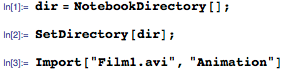#### Internal damping, basic equations

Damping in a mechanical system is a technique for lowering vibration. Damping almost always works for a non-rotating system. However, in the first half of the twentieth century many engineers learned the hard way that increasing the damping in rotating structures may lead to catastrophic failures.

When this phenomenon is studied, a system like that in the figure below is often used. A disc with mass m is mounted on a shaft with stiffness k and internal viscous damping ci. The shaft is subjected to a fictitious external damper with the damping constant ce. The rotor rotates with the angular velocity W. An external force F is acting on the mass: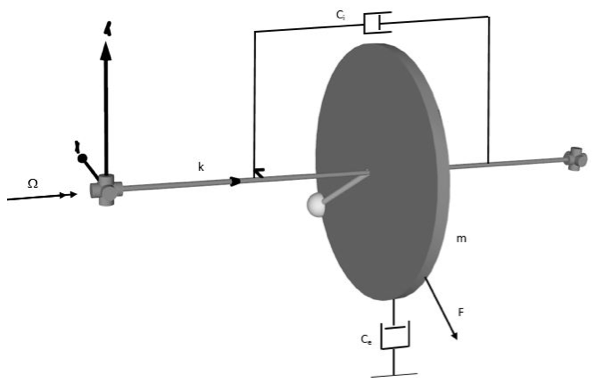Let the x-y-z coordinate system be fixed in space and the ξ-η-z coordinate system be fixed in the shaft, rotating with the angular velocity Ω. The transformation of the coordinate system between these two systems will be: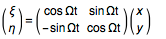The transformation of the forces will be: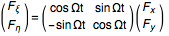The internal forces acting on the mass will be: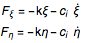Transforming both the forces and the deflections to the fixed coordinate: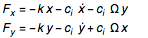The equation of motion for the system can now be written as: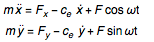The damping force, given by ceẋ and ceẏ, is assumed to act directly on the disc.

The equation of motion in matrix form: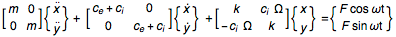Rewrite to the complex form and let: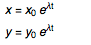Study the homogeneous part—the external force F is equal to zero: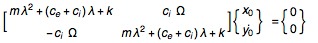The nonzero solution requires that the determinant is equal to zero or: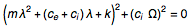This equation has a solution of the form λ = Κ + i ω. If Κ > 0, the motion is unstable. And if Κ < 0, the motion is stable. The stability threshold is obtained when Κ = 0, i.e. λ = i ω. The determinant can be written as: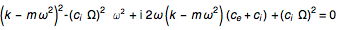Both the real and imaginary parts have to individually be zero. The real part gives: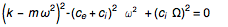And the imaginary part gives: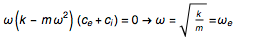Simplify the real part and use the solution for the imaginary part: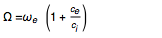In the world of vibration and rotor dynamics, this is a well-known relation. It follows from the latter equation that the motion will be stable up until a certain limit of the rotor’s rotational frequency. Above this limit, the motion will be unstable. Once it becomes unstable, it will whirl with a frequency equal to the eigenfrequency.

We may make the following observation: if the internal damping is much higher than external damping, i.e. the bearing damping, it is not wise to exceed the first resonance frequency. This is the reason why many electrical motors with high internal damping never pass the eigenfrequency. Note also that a rotor vibration with its (lowest) eigenfrequency, although the rotational frequency is different, is most likely unstable. This is a behavior that also yields from many other situations than internal damping instability.

#### Wolfram SystemModeler model

The SystemModeler model is built up with standard components and an Euler–Bernoulli beam. In this application, we use ten beam elements on each side. The resulting damping is Rayleigh damping and is proportional to the mass and the stiffness matrix. In this theoretical example, we need to have an external static force for the damping test and also a component for damping close to the mass as the external damping. In reality, external damping will include the bearings: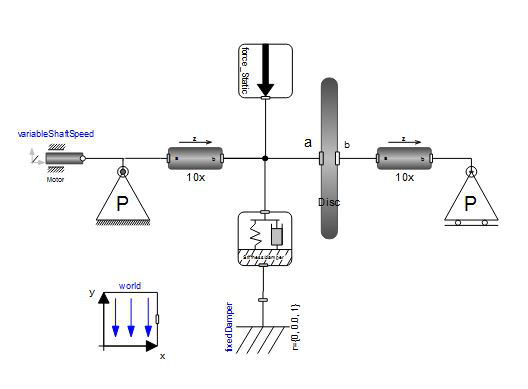The pinned supports are easily built up with just a few standard components: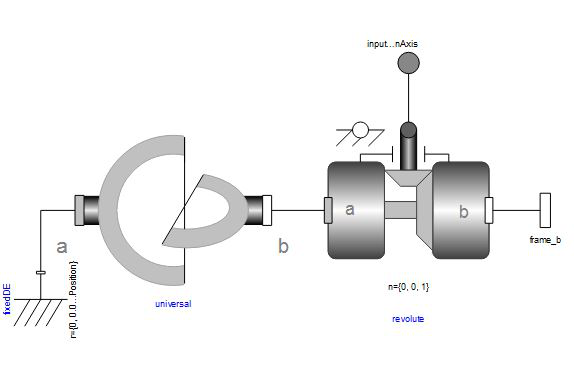Our first task is to determine the equivalent viscous damping coefficient due to Rayleigh damping. We do it by the same method as determining damping when it is measured out in the field. Load it and release it, and measure the damping and frequencies. The setup is shown in the following film clip:

The response in the vertical direction: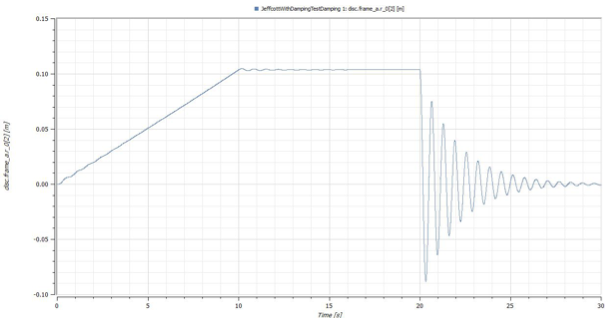The equivalent viscous damping constant for these P-P beams can now be determined by looking at how fast the vibrations’ amplitude decreases. If the amplitude for the first five peaks after the release at 20 s are taken into account, the equivalent viscous damping will be: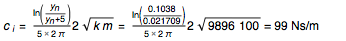Set the external damping to:

ce=100 Ns/m

The system will become unstable at approximately: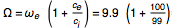≈20 rad/s(⟷ 200 seconds in the below graph).

The figure shows the deflection when running up from 0 to 30 rad/s during 300 s: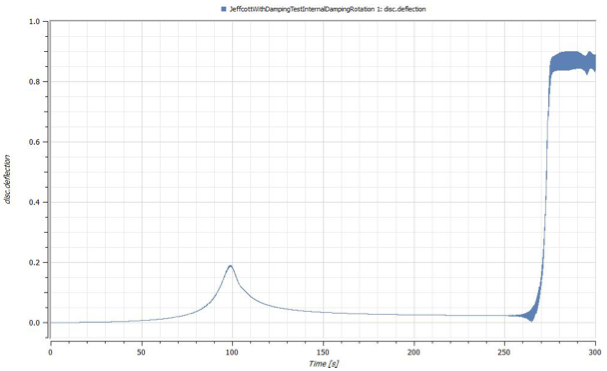As can be seen, the system starts to become unstable at 2.5 times the resonance frequency, exactly as the theory says. The instability in this simulation is fully developed at approximately 2.75 times resonance frequency.

An FFT on the vibration between 190–200 seconds (i.e. shaft speed 19–20 rad/s or approximately 3.1 Hz) looks like: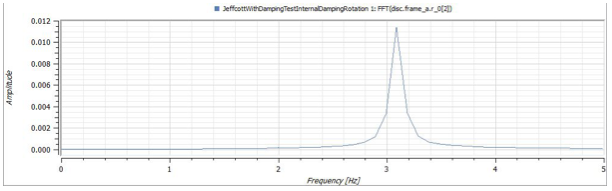As expected, the motion of the whirling shaft follows the shaft rotation. What happens with the vibration frequency when the system becomes unstable? That is the interesting part. In the figure below, the shaft rotates at 25 rad/s but the mass whirls at 9.9 rad/s, i.e. the first eigenfrequency. So the behavior is exactly as the theory predicts: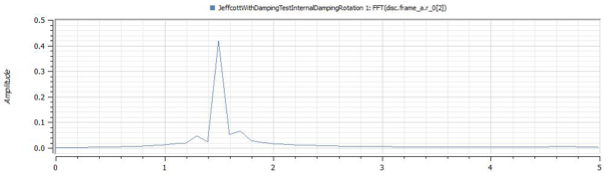#### Summary

SystemModeler is a powerful tool for studying advanced problems in rotating machinery. This example is nearly impossible for most commercial finite element software without a lot of extra work. With SystemModeler, it is straightforward.

We conclude by looking at a video depicting the system when the internal damping is present. Below, the first eigenfrequency, the imbalance, and the whirl are in phase (0–10 rad/s). Then they are out of phase at between 10–25 rad/s. And then the system is unstable and whirls at 10 rad/s: